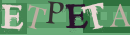1- University of Kharazmi , msaberi.khu@gmail.com
2- University of Kharazmi
Abstract:   (1159 Views)
many geomorphic landforms have fractal structures and their formation and transformation can be explained by mathematical relations. The purpose of this study is to identify and analyze the fractal behavior of landforms of macro geomorphologic regions of Iran,as well as studying and analyzing topographic and landform characteristics based on fractal relationships, and also, analyzing the characteristics of dominant geomorphologic processes based on the theory of fractals. In this study, the contour lines of different landforms of Iran (according to the territorial types) including mountains,hills, plateaus, Plain Domains, Fan Breakout, fan alluvial, for pixel sizes of 30,90,200 m, were drawn and their fractal dimension was estimated by using the box-counting method. The morphometric characteristics of the landforms and their fractal dimensions with indexes (max, mean and standard deviation) related to the five variables (height, gradient, profile curvature and planar curvature of the metric) were analyzed by Arc GIS software at each layer.After investigating their correlation with the fractal dimension, the regression analysis was performed binary and the relationship between the fractal dimension,topography, landforms and processes was analyzed. The fractal dimension has the highest correlation coefficient with the gradient and the standard deviation indices, and the lowest coefficient with the profile curvature and the mean index Moreover, for larger pixel sizes, the correlation coefficient decreases between the indices and the fractal dimension.This research can provide a ground for further research on fractal geometry in geography, geomorphology, geology, environment and other Earth sciences.

Type of Study: Applicable | Subject: GeomorphologyRights and permissionsThis work is licensed under a Creative Commons Attribution-NonCommercial 4.0 International License.